Publicité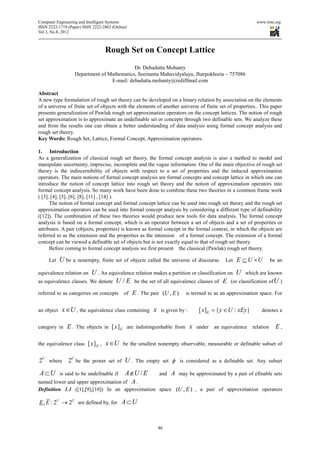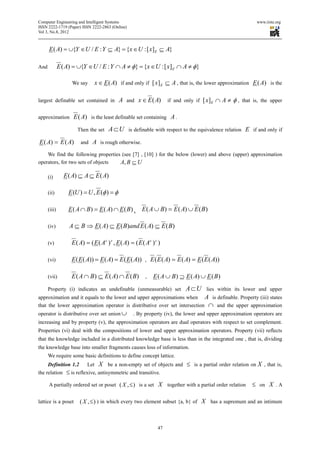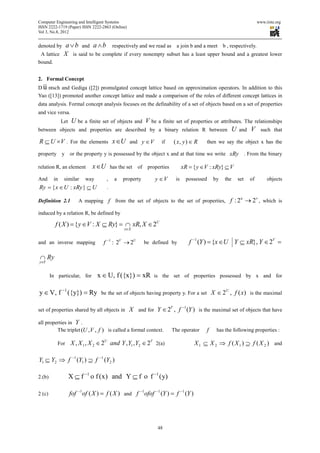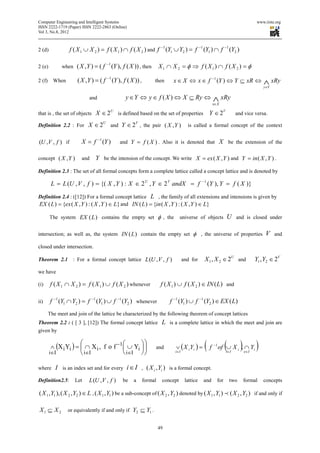Publicité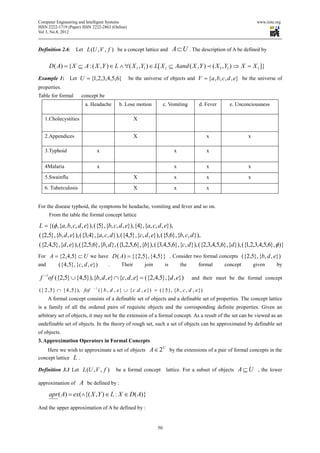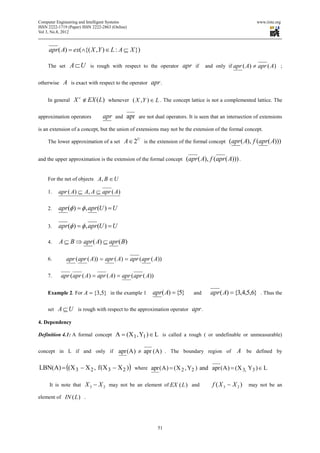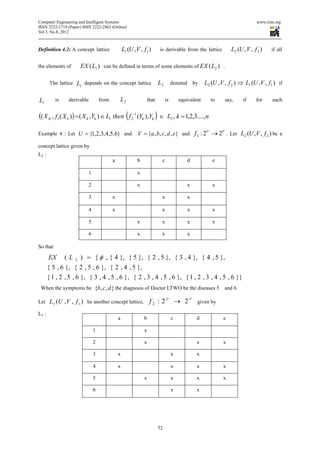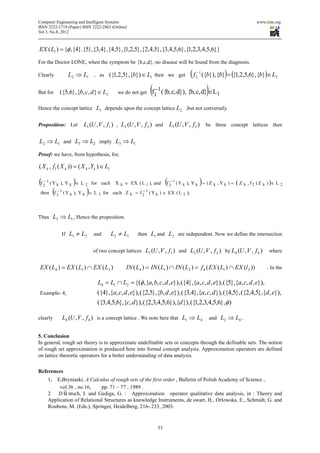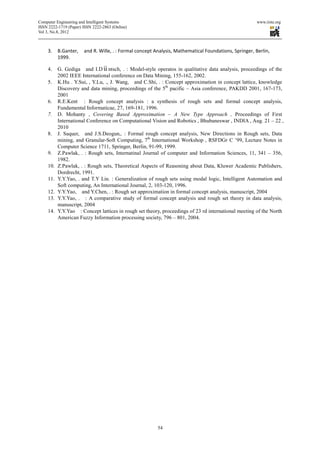Publicité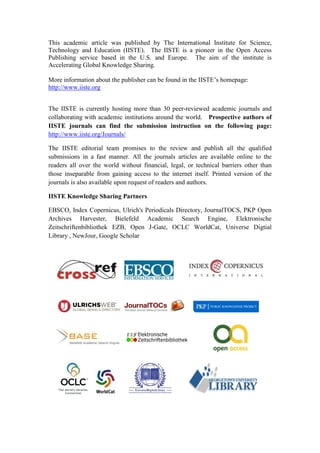Prochain SlideShare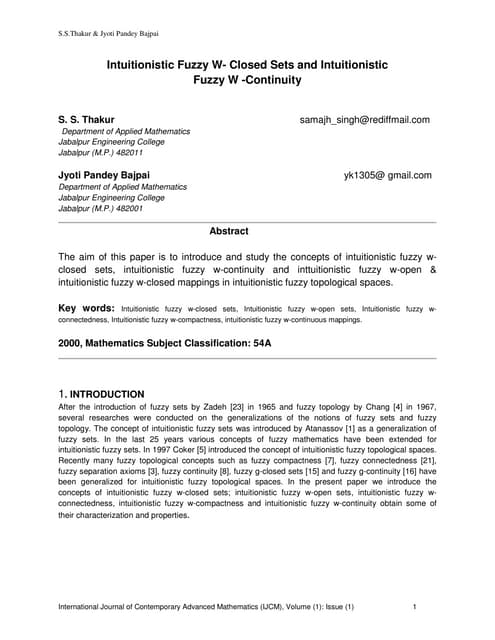Intuitionistic Fuzzy W- Closed Sets and Intuitionistic Fuzzy W -Continuity
Chargement dans ... 3
1 sur 10
Publicité

### Rough set on concept lattice

1. Computer Engineering and Intelligent Systems www.iiste.org ISSN 2222-1719 (Paper) ISSN 2222-2863 (Online) Vol 3, No.8, 2012 Rough Set on Concept Lattice Dr. Debadutta Mohanty Department of Mathematics, Seemanta Mahavidyalaya, Jharpokhoria – 757086 E-mail: debadutta.mohanty@rediffmail.com Abstract A new type formulation of rough set theory can be developed on a binary relation by association on the elements of a universe of finite set of objects with the elements of another universe of finite set of properties.. This paper presents generalization of Pawlak rough set approximation operators on the concept lattices. The notion of rough set approximation is to approximate an undefinable set or concepts through two definable sets. We analyze these and from the results one can obtain a better understanding of data analysis using formal concept analysis and rough set theory. Key Words: Rough Set, Lattice, Formal Concept, Approximation operators. 1. Introduction As a generalization of classical rough set theory, the formal concept analysis is aiso a method to model and manipulate uncertainty, imprecise, incomplete and the vague information. One of the main objective of rough set theory is the indiscernibility of objects with respect to a set of properties and the induced approximation operators. The main notions of formal concept analysis are formal concepts and concept lattice in which one can introduce the notion of concept lattice into rough set theory and the notion of approximation operators into formal concept analysis. So many work have been done to combine these two theories in a common frame work ( , , , , ,  ,  ). The notion of formal concept and formal concept lattice can be used into rough set theory and the rough set approximation operators can be used into formal concept analysis by considering a different type of definability (). The combination of these two theories would produce new tools for data analysis. The formal concept analysis is based on a formal concept, which is an operator between a set of objects and a set of properties or attributes. A pair (objects, properties) is known as formal concept in the formal context, in which the objects are referred to as the extension and the properties as the intension of a formal concept. The extension of a formal concept can be viewed a definable set of objects but is not exactly equal to that of rough set theory. Before coming to formal concept analysis we first present the classical (Pawlak) rough set theory. Let U be a nonempty, finite set of objects called the universe of discourse. Let E ⊆ U ×U be an equivalence relation on U . An equivalence relation makes a partition or classification on U which are known as equivalence classes. We denote U / E be the set of all equivalence classes of E (or classification of U ) referred to as categories on concepts of E . The pair (U , E ) is termed to as an approximation space. For an object x∈U , the equivalence class containing x is given by : [ x ]E = { y ∈ U : xEy} denotes a category in E . The objects in [ x] E are indistinguishable from x under an equivalence relation E , the equivalence class [ x] E , x∈U be the smallest nonempty observable, measurable or definable subset of 2U where 2U be the power set of U . The empty set φ is considered as a definable set. Any subset A ⊂U is said to be undefinable if A ∉U / E and A may be approximated by a pair of efinable sets named lower and upper approximation of A . Definition 1.1 (,,) In an approximation space (U , E ) , a pair of approximation operators E , E : 2U → 2U are defined by, for A ⊂ U 46
2. Computer Engineering and Intelligent Systems www.iiste.org ISSN 2222-1719 (Paper) ISSN 2222-2863 (Online) Vol 3, No.8, 2012 E ( A) = ∪{Y ∈ U / E : Y ⊆ A} = {x ∈ U : [ x ] E ⊆ A} And E ( A) = ∪{Y ∈U / E : Y ∩ A ≠ φ} = {x ∈U : [ x] E ∩ A ≠ φ} We say x ∈ E ( A) if and only if [ x ] E ⊆ A , that is, the lower approximation E ( A) is the largest definable set contained in A and x ∈ E (A) if and only if [ x ] E ∩ A ≠ φ, that is, the upper approximation E ( A) is the least definable set containing A . Then the set A ⊂U is definable with respect to the equivalence relation E if and only if E ( A) = E ( A) and A is rough otherwise. We find the following properties (see  ,  ) for the below (lower) and above (upper) approximation operators, for two sets of objects A, B ⊆ U (i) E ( A) ⊆ A ⊆ E ( A) (ii) E (U ) = U , E (φ ) = φ (iii) E ( A ∩ B ) = E ( A) ∩ E ( B ) , E ( A ∪ B) = E ( A) ∪ E ( B ) (iv) A ⊆ B ⇒ E ( A) ⊆ E ( B)and E ( A) ⊆ E ( B) (v) E ( A) = ( E ( A c ) c , E ( A) = ( E ( A c ) c ) (vi) E ( E ( A)) = E ( A) = E ( E ( A)) , E ( E ( A) = E ( A) = E ( E ( A)) (vii) E ( A ∩ B ) ⊆ E ( A) ∩ E ( B) , E ( A ∪ B ) ⊇ E ( A) ∪ E ( B ) Property (i) indicates an undefinable (unmeasurable) set A ⊂U lies within its lower and upper approximation and it equals to the lower and upper approximations when A is definable. Property (iii) states that the lower approximation operator is distributive over set intersection ∩ and the upper approximation operator is distributive over set union ∪ . By property (iv), the lower and upper approximation operators are increasing and by property (v), the approximation operators are dual operators with respect to set complement. Properties (vi) deal with the compositions of lower and upper approximation operators. Property (vii) reflects that the knowledge included in a distributed knowledge base is less than in the integrated one , that is, dividing the knowledge base into smaller fragments causes loss of information. We require some basic definitions to define concept lattice. Definition 1.2 Let X be a non-empty set of objects and ≤ is a partial order relation on X , that is, the relation ≤ is reflexive, antisymmetric and transitive. A partially ordered set or poset ( X , ≤ ) is a set X together with a partial order relation ≤ on X . A lattice is a poset ( X , ≤ ) ) in which every two element subset {a, b} of X has a supremum and an intimum 47
3. Computer Engineering and Intelligent Systems www.iiste.org ISSN 2222-1719 (Paper) ISSN 2222-2863 (Online) Vol 3, No.8, 2012 denoted by a∨b and a ∧b respectively and we read as a join b and a meet b , respectively. A lattice X is said to be complete if every nonempty subset has a least upper bound and a greatest lower bound. 2. Formal Concept D && ntsch and Gediga () promulgated concept lattice based on approximation operators. In addition to this u Yao () promoted another concept lattice and made a comparison of the roles of different concept lattices in data analysis. Formal concept analysis focuses on the definability of a set of objects based on a set of properties and vice versa. Let U be a finite set of objects and V be a finite set of properties or attributes. The relationships between objects and properties are described by a binary relation R between U and V such that R ⊆ U × V . For the elements x ∈U and y ∈ V if ( x, y ) ∈ R then we say the object x has the property y or the property y is possessed by the object x and at that time we write xRy . From the binary relation R, an element x ∈U has the set of properties xR = { y ∈V : xRy} ⊆ V And in similar way , a property y ∈V is possessed by the set of objects Ry = { x ∈ U : xRy } ⊆ U . Definition 2.1 A mapping f from the set of objects to the set of properties, f : 2 u → 2 v , which is induced by a relation R, be defined by f ( X ) = { y ∈V : X ⊆ Ry} = ∩ xR, X ∈ 2U x∈X and an inverse mapping f −1 : 2V → 2U be defined by f −1 (Y ) = {x ∈U Y ⊆ xR}, Y ∈ 2V = ∩ Ry y∈Y In particular, for x ∈ U, f ({x}) = xR is the set of properties possessed by x and for y ∈ V, f −1 ({y}) = Ry be the set of objects having property y. For a set X ∈ 2U , f ( x) is the maximal set of properties shared by all objects in X and for Y ∈ 2V , f −1 (Y ) is the maximal set of objects that have all properties in Y . The triplet (U , V , f ) is called a formal context. The operator f has the following properties : For X , X 1 , X 2 ∈ 2U and Y , Y1 , Y2 ∈ 2V 2(a) X 1 ⊆ X 2 ⇒ f ( X 1 ) ⊇ f ( X 2 ) and −1 −1 Y1 ⊆ Y2 ⇒ f (Y1 ) ⊇ f (Y2 ) 2.(b) X ⊆ f −1 o f ( x ) and Y ⊆ f o f −1 ( y) 2 (c) fof −1of ( X ) = f ( X ) and f −1ofof −1 (Y ) = f −1 (Y ) 48
4. Computer Engineering and Intelligent Systems www.iiste.org ISSN 2222-1719 (Paper) ISSN 2222-2863 (Online) Vol 3, No.8, 2012 2 (d) f ( X 1 ∪ X 2 ) = f ( X 1 ) ∩ f ( X 2 ) and f −1 (Y1 ∪ Y2 ) = f −1 (Y1 ) ∩ f −1 (Y2 ) 2 (e) when ( X , Y ) = ( f −1 (Y ), f ( X )) , then X1 ∩ X 2 = φ ⇒ f ( X1) ∩ f ( X 2 ) = φ 2 (f) When ( X , Y ) = ( f −1 (Y ), f ( X )) , then x∈ X ⇔ x∈ f −1 (Y ) ⇔ Y ⊆ xR ⇔ ∧ xRy y∈Y and y ∈ Y ⇔ y ∈ f ( X ) ⇔ X ⊆ Ry ⇔ ∧ xRy x∈X that is , the set of objects X ∈ 2U is defined based on the set of properties Y ∈ 2V and vice versa. Definition 2.2 : For X ∈ 2U and Y ∈ 2V , the pair ( X , Y ) is called a formal concept of the context (U ,V , f ) if X = f −1 (Y ) and Y = f ( X ) . Also it is denoted that X be the extension of the concept ( X , Y ) and Y be the intension of the concept. We write X = ex ( X , Y ) and Y = in ( X , Y ) . Definition 2.3 : The set of all formal concepts form a complete lattice called a concept lattice and is denoted by −1 L = L (U , V , f ) = {( X , Y ) : X ∈ 2 U , Y ∈ 2 V andX = f (Y ), Y = f ( X )} Definition 2.4 : () For a formal concept lattice L , the family of all extensions and intensions is given by EX ( L ) = {ex ( X , Y ) : ( X , Y ) ∈ L} and IN ( L ) = {in ( X , Y ) : ( X , Y ) ∈ L} The system EX (L ) contains the empty set φ , the universe of objects U and is closed under intersection; as well as, the system IN (L ) contain the empty set φ , the universe of properties V and closed under intersection. Theorem 2.1 : For a formal concept lattice L (U , V , f ) and for X 1 , X 2 ∈ 2U and Y1 , Y2 ∈ 2V we have (i) f ( X 1 ∩ X 2 ) = f ( X 1 ) ∪ f ( X 2 ) whenever f ( X 1 ) ∪ f ( X 2 ) ∈ IN ( L ) and ii) f −1 (Y1 ∩ Y2 ) = f −1 (Y1 ) ∪ f −1 (Y2 ) whenever f −1 (Y1 ) ∪ f −1 (Y2 ) ∈ EX ( L) The meet and join of the lattice be characterized by the following theorem of concept lattices Theorem 2.2 : ( [ 3 ], ) The formal concept lattice L is a complete lattice in which the meet and join are given by i∈I   i∈I    i∈I    ∧ (X i Yi ) =  ∩ X i , f o f −1  ∪ Yi   and ∨ ( X i Yi ) = i∈ I (f −1 ( of ∪ X i , ∩ Yi i∈ I ) e∈ I ) where I is an index set and for every i ∈ I , ( X i , Yi ) is a formal concept. Definition2.5: Let L (U , V , f ) be a formal concept lattice and for two formal concepts ( X 1 , Y1 ), ( X 2 , Y2 ) ∈ L , ( X 1 , Y1 ) be a sub-concept of ( X 2 , Y2 ) denoted by ( X 1 , Y1 ) p ( X 2 , Y2 ) if and only if X1 ⊆ X 2 or equivalently if and only if Y2 ⊆ Y1 . 49
5. Computer Engineering and Intelligent Systems www.iiste.org ISSN 2222-1719 (Paper) ISSN 2222-2863 (Online) Vol 3, No.8, 2012 Definition 2.6: Let L (U , V , f ) be a concept lattice and A ⊂ U . The description of A be defined by D ( A) = { X ⊆ A : ( X , Y ) ∈ L ∧ ∀( X 1 , Y1 ) ∈ L[ X 1 ⊆ Aand ( X , Y ) p ( X 1 , Y1 ) ⇒ X = X 1 ]} Example 1: Let U = {1, 2,3, 4,5,6} be the universe of objects and V = {a , b , c , d , e} be the universe of properties. Table for formal concept be a. Headache b. Lose motion c. Vomiting d. Fever e. Unconciousness 1.Cholecystities X 2.Appendices X x x 3.Typhoid x x x 4Malaria x x x x 5.Swainflu X x x x 6. Tuberculosis X x x For the disease typhoid, the symptoms be headache, vomiting and fever and so on. From the table the formal concept lattice L = {(φ , {a, b, c, d , e}), ({5}, {b, c, d , e}),{4}, {a, c, d , e}), ({2,5}, {b, d , e}), ({3,4}, {a, c, d }), ({4,5}, {c, d , e}), ({5,6}, {b, c, d }), ({2,4,5},{d , e}), ({2,5,6},{b, d }, ({1,2,5,6}, {b}), ({3,4,5,6}, {c, d }), ({2,3,4,5,6}, {d }), ({1,2,3,4,5,6}, φ )} For A = {2, 4,5} ⊂ U we have D ( A) = {{ 2,5}, {4,5}} . Consider two formal concepts ({2,5}, {b, d , e}) and ({4,5}, {c , d , e}) . Their join is the formal concept given by f −1of ({2,5} ∪ {4,5}),{b, d , e} ∩ {c, d , e} = ({2,4,5},{d , e}) and their meet be the formal concept −1 ({ 2 , 5 } ∩ { 4 , 5 }), fof ({ b , d , e } ∪ { c . d , e }) = ({ 5 }, { b , c , d , e }) A formal concept consists of a definable set of objects and a definable set of properties. The concept lattice is a family of all the ordered pairs of requisite objects and the corresponding definite properties. Given an arbitrary set of objects, it may not be the extension of a formal concept. As a result of the set can be viewed as an undefinable set of objects. In the theory of rough set, such a set of objects can be approximated by definable set of objects. 3. Approximation Operators in Formal Concepts Here we wish to approximate a set of objects A ∈ 2U by the extensions of a pair of formal concepts in the concept lattice L . Definition 3.1 Let L (U , V , f ) be a formal concept lattice. For a subset of objects A ⊆ U , the lower approximation of A be defined by : apr ( A) = ex(∧{( X , Y ) ∈ L : X ∈ D( A)} And the upper approximation of A be defined by : 50
6. Computer Engineering and Intelligent Systems www.iiste.org ISSN 2222-1719 (Paper) ISSN 2222-2863 (Online) Vol 3, No.8, 2012 apr ( A) = ex(∧{( X , Y ) ∈ L : A ⊆ X }) The set A ⊂U is rough with respect to the operator apr if and only if apr ( A) ≠ apr ( A) ; otherwise A is exact with respect to the operator apr . In general X c ∉ EX (L) whenever ( X , Y ) ∈ L . The concept lattice is not a complemented lattice. The approximation operators apr and apr are not dual operators. It is seen that an intersection of extensions is an extension of a concept, but the union of extensions may not be the extension of the formal concept. The lower approximation of a set A ∈ 2U is the extension of the formal concept (apr( A), f (apr( A))) and the upper approximation is the extension of the formal concept (apr ( A), f (apr ( A))) . For the net of objects A, B ∈ U 1. apr ( A) ⊆ A, A ⊆ apr ( A) 2. apr (φ ) = φ , apr (U ) = U 3. apr (φ ) = φ , apr (U ) = U 4. A ⊆ B ⇒ apr ( A) ⊆ apr ( B) 6. apr ( apr ( A)) = apr ( A) = apr ( apr ( A)) 7. apr ( apr ( A) = apr ( A) = apr ( apr ( A)) Example 2. For A = {3,5} in the example 1 apr( A) = {5} and apr ( A) = {3,4,5,6} . Thus the set A ⊆ U is rough with respect to the approximation operator apr . 4. Dependency Definition 4.1: A formal concept A = ( X 1 , Y1 ) ∈ L is called a rough ( or undefinable or unmeasurable) concept in L if and only if apr ( A ) ≠ apr ( A ) . The boundary region of A be defined by LBN(A) = ((X 3 − X 2 , f (X 3 − X 2 )) where apr ( A ) = ( X 2 , Y2 ) and apr ( A ) = ( X 3, Y3 ) ∈ L It is note that X 3 − X 2 may not be an element of EX (L ) and f ( X 3 − X 2 ) may not be an element of IN (L ) . 51
7. Computer Engineering and Intelligent Systems www.iiste.org ISSN 2222-1719 (Paper) ISSN 2222-2863 (Online) Vol 3, No.8, 2012 Definition 4.2: A concept lattice L1 (U ,V , f1 ) is derivable from the lattice L2 (U , V , f 2 ) if all the elements of EX ( L1 ) can be defined in terms of some elements of EX ( L2 ) . The lattice L1 depends on the concept lattice L2 denoted by L2 (U , V , f 2 ) ⇒ L1 (U ,V , f1 ) if L1 is derivable from L2 that is equivalent to say, if for each (( X K , f1 ( X k )) = ( X k ,Yk ) ∈ L1 then ( f 2−1 (Yk ),Yk ) ∈ L2 , k = 1,2,3...., n V = {a , b , c , d , e} and f 2 : 2 → 2 . Let L2 (U ,V , f 2 ) be a U V Example 4 : Let U = {1, 2,3, 4,5,6} and concept lattice given by L2 : a b c d e 1 x 2 x x x 3 x x x 4 x x x x 5 x x x x 6 x x x So that EX (L 2 ) = { φ , { 4 }, { 5 }, { 2 , 5 }, { 3 , 4 }, { 4 , 5 }, { 5 , 6 }, { 2 , 5 , 6 }, { 2 , 4 , 5 }, { 1 , 2 , 5 , 6 }, { 3 , 4 , 5 , 6 }, { 2 , 3 , 4 , 5 , 6 }, { 1 , 2 , 3 , 4 , 5 , 6 }} When the symptoms be {b , c , d } the diagnosis of Doctor LTWO be the diseases 5 and 6. Let L 1 (U , V , f 1 ) be another concept lattice, f2 : 2U → 2V given by L1 : a b c d e 1 x 2 x x x 3 x x x 4 x x x x 5 x x x x 6 x x 52
8. Computer Engineering and Intelligent Systems www.iiste.org ISSN 2222-1719 (Paper) ISSN 2222-2863 (Online) Vol 3, No.8, 2012 EX ( L1 ) = {φ ,{4}.{5},{3,4},{4,5},{1,2,5},{2,4,5},{3,4,5,6},{1,2,3,4,5,6}} For the Doctor LONE, when the symptom be {b,c,d}, no disease will be found from the diagnosis. Clearly L2 ⇒ L1 , as ({1,2,5},{b}) ∈ L1 then we get (f 2 −1 ) ({b}), {b} = ({1,2,5,6}, {b})∈ L2 But for ({5,6},{b, c, d } ∈ L2 we do not get (f1−1({b, c, d}), {b, c, d})∈L1 Hence the concept lattice L1 depends upon the concept lattice L 2 ,but not conversely. Proposition: Let L1 (U ,V , f1 ) , L2 (U , V , f 2 ) and L3 (U , V , f 3 ) be three concept lattices then L2 ⇒ L1 and L3 ⇒ L2 imply L3 ⇒ L1 Proof: we have, from hypothesis, for, ( X k , f1 ( X k )) = ( X k , Yk ) ∈ L1 (f 2− 1 ( Y k ), Y k )∈ L 2 for each X k ∈ EX ( L 1 ), and (f 2− 1 ( Y k ), Y k ) = ( Z k , Y k ) = ( Z k , f 2 ( Z k ) )∈ L 2 then (f 3 1 ( Y k ), Y k )∈ L 3 for each Z k = f 2 1 ( Y k ) ∈ EX ( L 2 ); − − Thus L3 ⇒ L1 , Hence the proposition. If L1 ≠ L2 and L2 ≠ L1 then L1 and L 2 are independent. Now we define the intersection of two concept lattices L1 (U ,V , f1 ) and L2 (U , V , f 2 ) by L4 (U , V , f 4 ) where EX ( L4 ) = EX ( L1 ) ∩ EX ( L2 ) IN ( L4 ) = IN ( L1 ) ∩ IN ( L2 ) = f 4 ( EX ( L1 ) ∩ EX (l 2 )) . In the L4 = L1 ∩ L2 = {(φ ,{a, b, c, d , e}), ({4},{a, c, d , e}), ({5},{a, c, d , e}), Example- 4, ({4},{a, c, d , e}), ({2,5},{b, d , e}), ({3,4},{a, c, d }), ({4,5}, ({2,4,5},{d , e}), ({3,4,5,6},{c, d }), ({2,3,4,5,6}),{d }), ({1,2,3,4,5,6},φ ) clearly L4 (U , V , f 4 ) is a concept lattice . We note here that L1 ⇒ L4 and L2 ⇒ L4 . 5. Conclusion In general, rough set theory is to approximate undefinable sets or concepts through the definable sets. The notion of rough set approximation is produced here into formal concept analysis. Approximation operators are defined on lattice theoretic operators for a better understanding of data analysis. References 1. E.Bryniaski, A Calculus of rough sets of the first order , Bulletin of Polish Academy of Science , vol.36 , no.16, pp. 71 – 77 , 1989 . 2 D && ntsch, I. and Gediga, G. : Approximation operator qualitative data analysis, in : Theory and u Application of Relational Structures as knowledge Instruments, de swart, H., Orlowska, E., Schmidt, G. and Roubens, M. (Eds.), Springer, Heidelberg, 216- 233, 2003. 53
9. Computer Engineering and Intelligent Systems www.iiste.org ISSN 2222-1719 (Paper) ISSN 2222-2863 (Online) Vol 3, No.8, 2012 3. B.Ganter, and R. Wille, . : Formal concept Analysis, Mathematical Foundations, Springer, Berlin, 1999. 4. G. Gediga and I.D && ntsch, . : Model-style operatos in qualitative data analysis, proceedings of the u 2002 IEEE International conference on Data Mining, 155-162, 2002. 5. K.Hu . Y.Sui, , Y.Lu, ., J. Wang, and C.Shi, . : Concept approximation in concept lattice, knowledge Discovery and data mining, proceedings of the 5th pacific – Asia conference, PAKDD 2001, 167-173, 2001 6. R.E.Kent : Rough concept analysis : a synthesis of rough sets and formal concept analysis, Fundamental Informaticae, 27, 169-181, 1996. 7. D. Mohanty , Covering Based Approximation – A New Type Approach , Proceedings of First International Conference on Computational Vision and Robotics , Bhubaneswar , INDIA , Aug. 21 – 22 , 2010 8. J. Saquer, and J.S.Deogun,. : Formal rough concept analysis, New Directions in Rough sets, Data mining, and Granular-Soft Computing, 7th International Workshop , RSFDGr C ’99, Lecture Notes in Computer Science 1711, Springer, Berlin, 91-99, 1999. 9. Z.Pawlak, . : Rough sets, Internatinal Journal of computer and Information Sciences, 11, 341 – 356, 1982. 10. Z.Pawlak, . : Rough sets, Theoretical Aspects of Reasoning about Data, Kluwer Academic Publishers, Dordrecht, 1991. 11. Y.Y.Yao, . and T.Y Lin. : Generalization of rough sets using modal logic, Intelligent Automation and Soft computing, An International Journal, 2, 103-120, 1996. 12. Y.Y.Yao, and Y.Chen, . : Rough set approximation in formal concept analysis, manuscript, 2004 13. Y.Y.Yao, . : A comparative study of formal concept analysis and rough set theory in data analysis, manuscript, 2004 14. Y.Y.Yao : Concept lattices in rough set theory, proceedings of 23 rd international meeting of the North American Fuzzy Information processing society, 796 – 801, 2004. 54